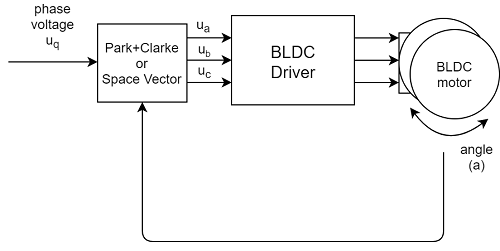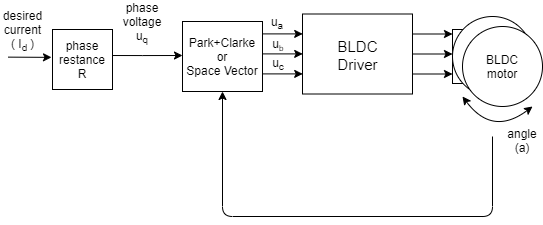# Torque control using voltage

This torque control approach allows you to run the BLDC motor as it is simple DC motor, where you set the target voltage Uq to be set to the motor and the FOC algorithm calculates the necessary phase voltages ua ,ub and uc for smooth operation. This mode is enabled by:

``````// voltage torque control mode
motor.torque_controller = TorqueControlType::voltage;
``````

## How does it work exactlyThe voltage control algorithm reads the angle a from the position sensor and the gets target Uq voltage value from the user and using the FOC algorithm sets the appropriate ua, ub and uc voltages to the motor. FOC algorithm ensures that these voltages generate the magnetic force in the motor rotor exactly with 90 degree offset from its permanent magnetic field, which guarantees maximal torque, this is called commutation.

The assumption is that the torque generated in the motor is proportional the voltage as Uq set buy user. Maximal torque corresponds to the maximal Uq which is conditioned by the power supply voltage available, and the minimal torque is of course for Uq = 0.

If the user provides the phase resistance value of the motor, the user can set the desired current Id and the library will automatically calculate the appropriate voltage Uq. This can be done either through the constructor for example

``````// BLDCMotor(pole pair number, phase resistance)
BLDCMotor motor = BLDCMotor( 11, 2.5 );
``````

or just setting the parameter:

``````motor.phase_resistance = 2.5; // ex. 2.5 Ohms
``````⚠️ The resulting current in the motor can, in some cases, be higher than the desired current Id but the order of the magnitude should be preserved.

For more info about the theory of the torque control check the section Digging deeper section or go directly to torque control theory.

## Configuration parameters

This control loop is very basic and it doesn’t really have any configuration parameters.

## Torque control example code

A simple example of the voltage based torque control and setting the target current by serial command interface.

``````#include <SimpleFOC.h>

// BLDC motor & driver instance
BLDCMotor motor = BLDCMotor(11);
BLDCDriver3PWM driver = BLDCDriver3PWM(9, 5, 6, 8);

// encoder instance
Encoder encoder = Encoder(2, 3, 500);
// channel A and B callbacks
void doA(){encoder.handleA();}
void doB(){encoder.handleB();}

// instantiate the commander
Commander command = Commander(Serial);
void doTarget(char* cmd) { command.variable(&motor.target, cmd); }

void setup() {

// initialize encoder sensor hardware
encoder.init();
encoder.enableInterrupts(doA, doB);
// link the motor to the sensor

// driver config
// power supply voltage [V]
driver.voltage_power_supply = 12;
driver.init();

// set the torque control type
motor.phase_resistance = 12.5; // 12.5 Ohms
motor.torque_controller = TorqueControlType::voltage;
// set motion control loop to be used
motor.controller = MotionControlType::torque;

// use monitoring with serial
Serial.begin(115200);
// comment out if not needed
motor.useMonitoring(Serial);

// initialize motor
motor.init();
// align sensor and start FOC
motor.initFOC();

Serial.println(F("Set the target current using serial terminal:"));
_delay(1000);
}

void loop() {

// main FOC algorithm function
motor.loopFOC();

// Motion control function
motor.move();

// user communication
command.run();
}
``````